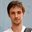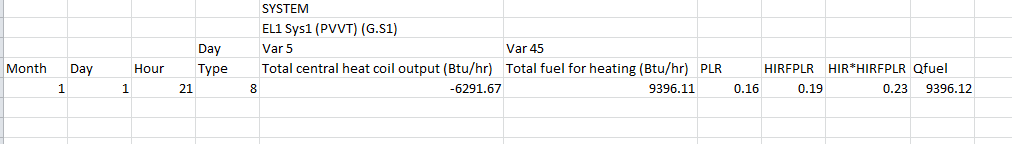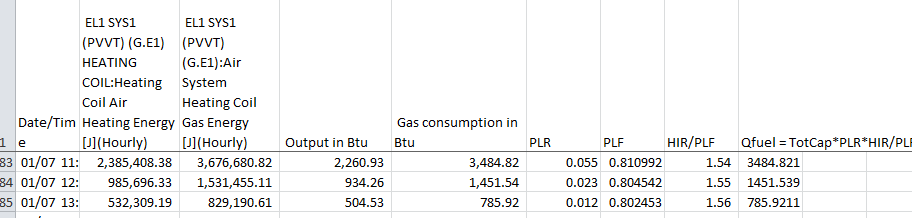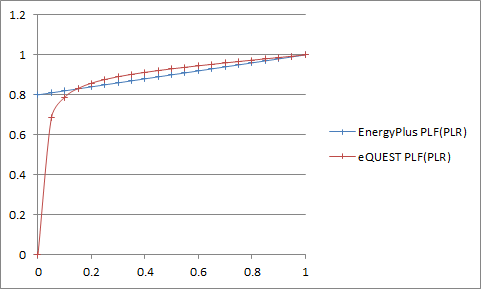Question-and-Answer Resource for the Building Energy Modeling Community
Get started with the Help page

# Furnace Efficiency DOE-2/EnergyPLusIn the EnergyPlus documentation, for the description of the part-load fraction performance curve for the furnaces, it says: "This [...] performance curve [...] parameterizes the variation of gas consumption rate by the heating coil as a function of the part load ratio [...]. For any simulation timestep, the nominal gas consumption rate (heating load/burner efficiency) is divided by the part-load fraction (PLF) if a part-load curve has been defined."

DOE-2 calculates the HIR-op as the product of the rated HIR and the HIR-FPLR(PLR).

I compared the default (when using a template) EnergyPlus performance curve for the PLF and the default curve for HIR-FPLR(PLR) in eQUEST. They are pretty pretty similar! Which makes me thinks that we can consider that PLF = HIR-FPLR(PLR).

The HIR for a particular time step is ratio the of fuel used to the heating energy produced.

According to the DOE-2 definition we have: Conso-op = f(Conso-rated * HIR-FPLR(PLR))

According to the EnergyPlus definition we have: Conso-op = f(Conso-rated / PLF)

Which is not the same thing and makes me think that PLF <> HIR-FPLR(PLR) ... Am I missing something?

edit retag close merge delete

Sort by » oldest newest most votedA little update as it might be useful for other users.

Neal's answer made sense the way he presented it. However, it didn't quite add up with the data I had. To find out what the calculation is in both cases, I generated hourly reports in both eQUEST and EnergyPlus. Here is what I found out:

## Calculation of the fuel consumption in DOE-2:

• Calculation of the PLR: PLR = (Load for the hour) / (Total Furnace Capacity)
• Use the PLR to get the HIR f(PLR) using the performance curve attached to the system
• Calculation of the operational HIR: HIR-op = HIR f(PLR) * HIR-rated
• Fuel consumption: Qfuel = (Total Furnace Capacity) * HIR-op (eq. 1)

Here is an excerpt from an hourly report from eQUEST that illustrate the calculation:In this example, the total furnace capacity is 39988 Btu/h and the rated HIR is 1.25.

## Calculation of the fuel consumption in EnergyPlus:

• Calculation of the PLR: PLR = (Load for the hour) / (Total Furnace Capacity)
• Use the PLR to get the PLF f(PLR) using the performance curve attached to the system
• Fuel consumption: Qfuel = (Total Furnace Capacity) * PLR * HIR-rated / PLF (eq. 2)

Here is an excerpt from an hourly report from EnergyPlus that illustrate the calculation:In this example, the total furnace capacity is 39988 Btu/h and the rated HIR is 1.25.

## Comparison:

Then, if we look at (eq. 1) and (eq. 2):

• HIR f(PLR) * HIR-rated = PLR * HIR-rated / PLF
• Which simplifies to HIR f(PLR) = PLR / PLF
• Thus PLF = PLR / HIR f(PLR)

In conclusion PLF and HIR f(PLR) are not quite the same.

Using this relation I converted the curve used in eQUEST (by default) to the match the PLF used in EnergyPlus.

• EnergyPlus PLF = f(PLR) is the default curve used in EnergyPlus
• eQUEST PLF = f(PLR) is the default curve used in eQUEST converted to the EnergyPlus "format".more

This makes sense to me. I couldn't remember if PLR was implicit in the HIR curve in DOE-2, or accounted for separately.

1

Amazing post! I remember scratching my head about this when I first started using e+ / os, I stopped using it for a while and recently started back up and encountered the exact same issue! This post refreshed my memory. Thanks so much!

The HIR in DOE-2 is the inverse of the heating efficiency (eff).

In DOE-2 the calculated efficiency is: 1/(HIR*HIR-FPLR(PLR))

In EnergyPlus the calculated efficiency is: eff/PLF

So if eff = 1/HIR, then PLF and HIR-FPLR(PLR) are the same.

more